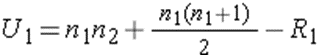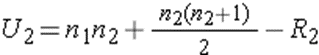# Mann-Whitney U test: hypotheses and example

## What is the Mann-Whitney U test?

The Mann-Whitney U test, also known as the Wilcoxon Rank Sum test, is a nonparametric statistical test used to compare two samples or groups.

The Mann-Whitney U test assesses whether two sampled groups are likely to come from the same population and essentially asks; Do these two populations have the same shape with respect to their data? In other words, we want evidence about whether the groups are drawn from populations with different levels of a variable of interest. It follows that the assumptions of a Mann-Whitney U-test are:

• The null hypothesis (H0) is that the two populations are equal.
• The alternative hypothesis (H1) is that the two populations are not equal.

Some researchers interpret this as a comparison of the medians between the two populations (in contrast, parametric tests compare the means between two independent groups). In some situations, where the data is of similar shape (see assumptions), this is valid – but it should be noted that the medians are not actually involved in the calculation of the Mann-Whitney U-test statistic. Two groups could have the same median and be significantly different according to the Mann-Whitney U test.

## When to use the Mann-Whitney U test

Nonparametric tests (sometimes called “distribution-free tests”) are used when you assume that the data from your populations of interest do not have a normal distribution. You can think of Mann Whitney’s U-test as analogous to the unpaired Student’s t-test, which you would use assuming your two populations are normally distributed, as defined by their means and standard deviation (the parameters of the distributions).

Figure 1: Normal distribution versus asymmetric distribution

The Mann-Whitney U test is a common statistical test used in many fields, including economics, biological sciences, and epidemiology. It is particularly useful when evaluating the difference between two independent groups with a small number of individuals in each group (usually less than 30), which are not normally distributed, and where the data is continuous. If you want to compare more than two groups with skewed data, a one-way Kruskal-Wallis analysis of variance (ANOVA) should be used.

Mann-Whitney U-test assumptions

Some key assumptions for the Mann-Whitney U test are detailed below:

• The variable compared between the two groups must be continued (able to take any number within a range – eg age, weight, height or heart rate). Indeed, the test is based on the ranking of the observations in each group.
• The data is assumed to take a not normal, or asymmetric distribution. If your data is normally distributed, the unpaired Student’s t-test should be used to compare the two groups instead.
• Although the data of both groups are not assumed to be normal, the data are assumed to be similar in shape through both groups.
• Data should be two randomly selected independent samples, which means that the groups have no relationship to each other. If the samples are paired (eg, two measurements from the same group of participants), a paired-samples t-test should be used instead.
• Sufficient sample size is needed for a valid test, usually more than 5 observations in each group.

## Mann-Whitney U-test example

Consider a randomized controlled trial evaluating a new antiretroviral therapy for HIV. A pilot trial randomly assigned participants to treated or untreated groups (N = 14). We want to assess the viral load (amount of virus per milliliter of blood) in the treated groups compared to the untreated groups. In practice, a Mann-Whitney U-test would be easily and quickly calculated using statistical software such as SPSS or Stata, but the steps are described below.

The data is shown below:

 Treaty 540 670 1000 960 1200 4650 4200 Untreated 5000 4200 1300 900 7400 4500 7500

These data are both skewed with a sample size of n = 7 in each treatment arm, and therefore a nonparametric test is appropriate. Before calculating the test, we choose a level of significance (usually α = 0.05). The first step is to assign ranks to the values ​​of the full sample (the two treatment groups combined) in order from smallest to largest. We can then generate a test statistic based on the ranks.

The table below shows the viral load values ​​in the treated and untreated groups ranked from smallest to largest, along with the summed ranks of each group:

 Viral load (treated) Viral load (untreated) Rank (processed) Rank (untreated) 540 1 670 2 900 3 960 4 1000 5 1200 6 1300 seven 4200 8 4500 9 4650 ten 5000 11 6100 12 7400 13 7500 14 R1=36 R2=69

After summing the ranks of each group, the Mann-Whitney U-test statistic is selected as the smallest of the following two calculated U values:Where we let 1 denote the treated group and 2 denote the untreated group (naming the groups is arbitrary), where n1 and n2 are the number of participants and where R1 and R2 are the sums of the ranks in the treated and untreated groups , respectively . In this example, U1=41 and U2=8. We therefore select U=8 as the test statistic.

## Normal approximation

There are situations where the sample size may be too large for the reference table to be used to calculate the exact probability distribution – in which case we can use a normal approximation instead. Since U is found by summing independent, similarly distributed random samples, the central limit theorem applies when the sample is large (usually >20 in each group). The standard deviation of the sum of the ranks can be used to generate a z-statistic and a significance value generated in this way. If the null hypothesis is true, the distribution of U approximates a normal distribution.

Next, we determine a “critical value” of U with which to compare our calculated test statistic, which we can do by using a critical value reference table and using our sample sizes (n=7 in both groups ) and the two-sided significance level (α=0.05).

In our current example, the critical value can be determined from the reference table as 8. Finally, we can use it to accept or reject the null hypothesis using the following decision rule: Reject H0 if U ≤ 8 .

Since our U statistic equals the critical value, we can reject the null hypothesis that the two groups are equal and accept the alternative hypothesis that there is evidence for a difference in viral load between the treated groups. with the new therapy compared to untreated.

Elliot McClenaghan is arresearcher in epidemiology and medical statistics at the London School of Hygiene & Tropical Medicine